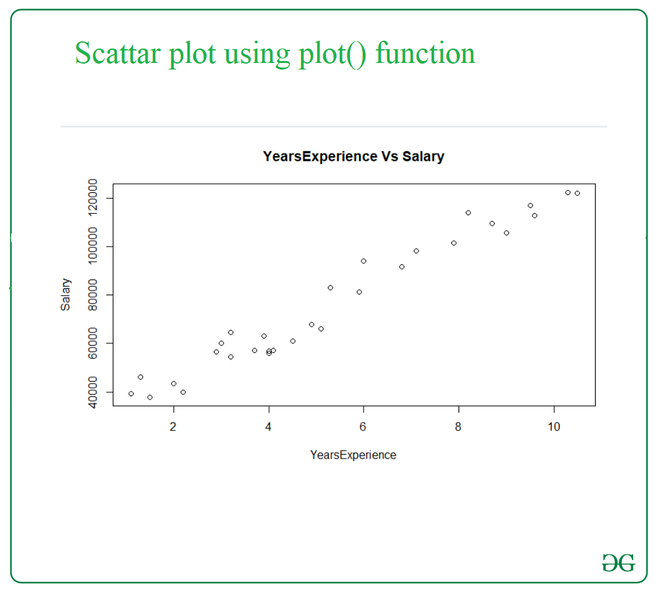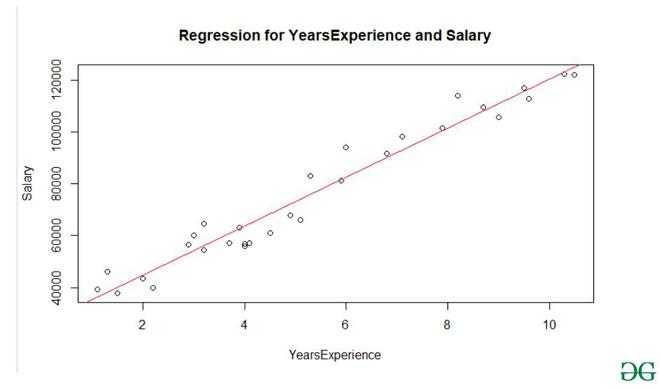# How to Create a Scatterplot with a Regression Line in R?

• Difficulty Level : Basic
• Last Updated : 17 Feb, 2021

A scatter plot uses dots to represent values for two different numeric variables. Scatter plots are used to observe relationships between variables. A linear regression is a straight line representation of relationship between an independent and dependent variable. In this article, we will discuss how a scatter plot with linear regression can be drafted using R and its libraries.

A scatter plot can be used to display all possible results and a linear regression plotted over it can be used to generalize common characteristics or to derive maximum points that follow up a result. Here we will first discuss the method of plotting a scatter plot and then draw a linear regression over it.

Used dataset: Salary_Data.xls

In R, function used to draw a scatter plot of two variables is plot() function which will return the scatter plot.

Syntax: plot(x, y, main, xlab, ylab, xlim, ylim, axes)

Parameters:-

• x- is the data set whose values are the horizontal coordinates.
• y- is the data set whose values are the vertical coordinates.
• main- is the tile of the graph.
• xlab- is the label on the horizontal axis.
• ylab- is the label on the vertical axis.
• xlim- is the limits of the values of x used for plotting.
• ylim- is the limits of the values of y used for plotting.
• axes- indicates whether both axes should be drawn on the plot.

Return:-

A 2-Dimension scatter plot.

Program:

## R

 `library``(readxl) ` ` `  `# import data ` `Salary_Data <- ``read_excel``(``"Salary_Data.xls"``) ` ` `  `# plot scatter plot ` `plot``(Salary_Data\$YearsExperience,Salary_Data\$Salary, ` `     ``main=``'YearsExperience Vs Salary'``, ` `     ``xlab=``'YearsExperience'``, ylab=``'Salary'``) `

Output:A regression line is a straight line that describes how a response variable y(Dependent variable) changes as an explanatory variable x(Independent)changes. This is used to predict the value of y for a given value of x.

For drawing regression line we need two functions:

• abline() function is used to add one or more straight lines through the current plot

Syntax: abline(a=NULL, b=NULL, h=NULL, v=NULL, …)

Parameters:
a, b: It specifies the intercept and the slope of the line
h: specifies y-value for horizontal line(s)
v: specifies x-value(s) for vertical line(s)

Returns: a straight line in the plot

• lm() function which stands for linear model,” function can be used to create a simple regression model.

Syntax: lm(formula,data)

Parameters:

• the formula- is a symbol presenting the relation between x and y.
• data- is the vector on which the formula will be applied.

Returns:

The relationship line of x and y.

Program:

## R

 `library``(readxl) ` ` `  `# import data ` `Salary_Data <- ``read_excel``(``"Salary_Data.xls"``) ` ` `  `# plot a scatter plot ` `plot``(Salary_Data\$YearsExperience,Salary_Data\$Salary, ` `     ``main=``'Regression for YearsExperience and Salary'``, ` `     ``xlab=``'YearsExperience'``,ylab=``'Salary'``) ` ` `  `# plot a regression line ` `abline``(``lm``(Salary~YearsExperience,data=Salary_Data),col=``'red'``) `

Output:My Personal Notes arrow_drop_up
Recommended Articles
Page :